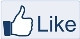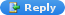# IMAGE PROCESSING

" Two roads diverged in a wood, and I,
I took the one less traveled by,
And that has made all the difference "-Robert Frost

### Matlab code: Histogram equalization without using histeq function

It is the re-distribution of gray level values uniformly. Let’s consider a 2 dimensional imagewhich has values ranging between 0 and 255.

MATLAB CODE:

``````

numofpixels=size(GIm,1)*size(GIm,2);

figure,imshow(GIm);

title('Original Image');
``````

``````

HIm=uint8(zeros(size(GIm,1),size(GIm,2)));

freq=zeros(256,1);

probf=zeros(256,1);

probc=zeros(256,1);

cum=zeros(256,1);

output=zeros(256,1);

%freq counts the occurrence of each pixel value.

%The probability of each occurrence is calculated by probf.

for i=1:size(GIm,1)

for j=1:size(GIm,2)

value=GIm(i,j);

freq(value+1)=freq(value+1)+1;

probf(value+1)=freq(value+1)/numofpixels;

end

end

sum=0;

no_bins=255;

%The cumulative distributionprobability is calculated.

for i=1:size(probf)

sum=sum+freq(i);

cum(i)=sum;

probc(i)=cum(i)/numofpixels;

output(i)=round(probc(i)*no_bins);

end

for i=1:size(GIm,1)

for j=1:size(GIm,2)

HIm(i,j)=output(GIm(i,j)+1);

end

end

figure,imshow(HIm);

title('Histogram equalization');
``````

``````

%The result is shown in the form of a table

figure('Position',get(0,'screensize'));

dat=cell(256,6);

for i=1:256

dat(i,:)={i,freq(i),probf(i),cum(i),probc(i),output(i)};

end

columnname =   {'Bin', 'Histogram', 'Probability', 'Cumulative histogram','CDF','Output'};

columnformat = {'numeric', 'numeric', 'numeric', 'numeric', 'numeric','numeric'};

columneditable =  [false false false false false false];

t = uitable('Units','normalized','Position',...

[0.1 0.1 0.4 0.9], 'Data', dat,...

'ColumnName', columnname,...

'ColumnFormat', columnformat,...

'ColumnEditable', columneditable,...

'RowName',[]);

subplot(2,2,2); bar(GIm);

title('Before Histogram equalization');

subplot(2,2,4); bar(HIm);

title('After Histogram equalization');
```

```

Here is a simple Version of Histogram Equalization MATLAB CODE:
``````

%Read a grayscale Image or a matrix mxn

figure,imshow(A);

%Specify the bin range[0 255]

bin=255;

%Find the histogram of the image.

Val=reshape(A,[],1);

Val=double(Val);

I=hist(Val,0:bin);

%Divide the result by number of pixels

Output=I/numel(A);

%Calculate the Cumlative sum

CSum=cumsum(Output);

%Perform the transformation S=T(R) where S and R in the range [ 0 1]

HIm=CSum(A+1);

%Convert the image into uint8

HIm=uint8(HIm*bin);

figure,imshow(HIm);
``````Like "IMAGE PROCESSING" pageAnonymous said...its nice that this space provides output too..and most importantly..the code works!!unlike most of the other sites!
-thank u soo much for this code.

sầu_đông said...good, very good

cool_images said...its last part is not working 3rd fig does not plot.ERROR::::bar must be 2 -D;
help me out

Unknown said...A graphical representation which shows a visual impression of the distribution of data termed as Histogram . Histograms consists of tabular frequencies which are shown as adjacent rectangles, with an area equal to the frequency of the observations in the interval.

Unknown said...@cool_images

just change the original image to gray-scale value, because the error occur and mention that only 2D

i had change in the end of the code as follows:

GIm1=rgb2gray(GIm);
subplot(2,2,2);
bar(GIm1);

title('Before Histogram equalization');
subplot(2,2,4);
bar(HIm);
title('After Histogram equalization');

Unknown said...sumi said...can i know, what is that mean with 1 and 2 in this code?
numofpixels=size(GIm,1)*size(GIm,2);
thanks.. ^^

Aaron Angel said...@sumi

it represents the dimensions. For a matrix, 1 represents rows and 2 represents columns.

Megha Atwal said...This is very nice tutorial here. Can you provide me with the code for CLAHE.

Khánh Thịnh said...Is there a bit problem with code, because max value of uint8 is 255, then
freq(value+1)=freq(value+1)+1;
cannot apply for the case value = 255. Further more, you set max gray value is 255, it is only right in almost case, not all case.

Aaron Angel said...@socksau

In matlab, the array range can start from 1. So the actual range 0 to 255 is mapped as 1 to 256.

Unknown said...can anybody give me code of face recognition system using k-means clustering algorithm and PCA .

Unknown said...After changing image to grayscale also, the last output is not working.. uitable is showing as unrecognised parameter : units.

manoj said...@Aaron Angel 1 means rows and 2 means columns of the image

Unknown said...i really doint understand how the freq funtion works someone care to explain?

Unknown said...whether histogram functions can be applied to rgb image or only to gray scale???

kassambe said...nice, could you generalize median filter to any size of the window?

Unknown said...I had the same problem with Rohit Ramesh (April 4, 2013). The 3rd figure shows nothing and the error as below:

Error using uitable
Object Name : uitable
Property Name : 'Units'.

Error in uitable (line 52)
thandle = builtin('uitable', varargin{:});

Error in territory7 (line 46)
t = uitable('Units','normalised','Position',...

සඳූ said...Love this blog. :) Good stuff! Keep it up!

Unknown said...tejas said...CAN U GIVE ME CODE FOR COMPARING TWO IMAGES IN MATLAB I WANT TO DETECT FAULT IN FABRIC

Unknown said...Can you give me code for IMAGE FUSION using Improved Synthetic Variable Ratio method? Please sir...

Unknown said...freq=zeros(256,1);

probf=zeros(256,1);

probc=zeros(256,1);

cum=zeros(256,1);

output=zeros(256,1);
what is the meaning of each line above??, please quick answer :) :)

Aaron Angel said...@ahmed ali

Pre-allocation of the vectors or 1D arrays. The gray level is[0 255] so there are 256 elements in total.Anonymous said...how to write a function to equilize a 100-by-100 image of normal random numbers with mean 128 and std-dev of 50.

Unknown said...can anybody provide full coding for image enhancement using histogram equalizatio..its urgent

Unknown said...histogram are also found different from that obtained from imhist(histeq(HIm))

Unknown said...How do i get the rgb for the pic?

Unknown said...its amazing; help us with a similar code for bi-histogram equalization also

I think there is an error with code "freq(value+1)=freq(value+1)+1" for the case value=255; when value=255, freq(value+1),that is, freq(256) cannot count correctly. freq(256) always gets "0" regardless of how i and j vary, instead freq(255) counts correctly. You may check the workspace for value of freq and compare the values of freq with that from the function imhist in Matlab.

Unknown said...This blog awesome and i learn a lot about programming from here.The best thing about this blog is that you doing from beginning to experts level.

Love from

Unknown said...Can i get a matlab code for multi peak generalized histogram equalisation?

Anu G@rg said...this blog is just awesome :)

Unknown said...i need program for comparing two images in face detection

Olivier Rukundo, PhD said...This code does NOT work. Yes, because the newest Matlab version RECOMMEND the use of HISTOGRAM instead of HIST (see: I=hist(Val,0:bin ). And, by using I = histogram (Val, 0:bin) you get the following error:

Undefined operator '/' for input arguments of type 'matlab.graphics.chart.primitive.Histogram'.

Error in histeq_test (line 15)
Output = I/numel(A);

By using I = histogram(Val,0:bin); and Output = double(I) /numel(A); The new error becomes:

Index exceeds matrix dimensions.

Error in histeq_test (line 21)
HIm = CSum(A + 1);

I therefore request the writer of this code to revise it, accordingly.

Unknown said...hello every one.... i need matlab code for adaptive contrast stretching without using of any built in matlab function.can any one help?

Unknown said...i like it its the simplest code i see it

Unknown said...@Aqeel Al-Surmi
It still doesnt work

inventormaalg said...thanks verey good code